# 方法

Hurst系数 （H） 是长期依赖的特征参数，与 FD （_FD + H = 2_）有关。 R / S分析是数据建模的核心工具。

H <0.5表示均值回复

H> 0.5表示趋势序​​列，并且

H = 0.5表示随机游走。

GARCH 模型的一个关键限制 是对其参数施加非负约束，以确保条件方差的正性。这样的约束会给估计GARCH 模型带来困难 。

# 数据挖掘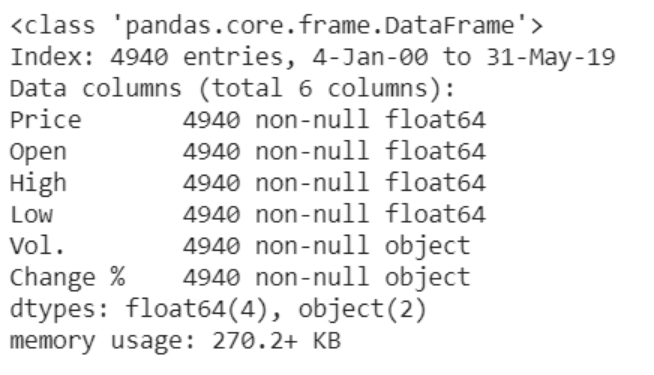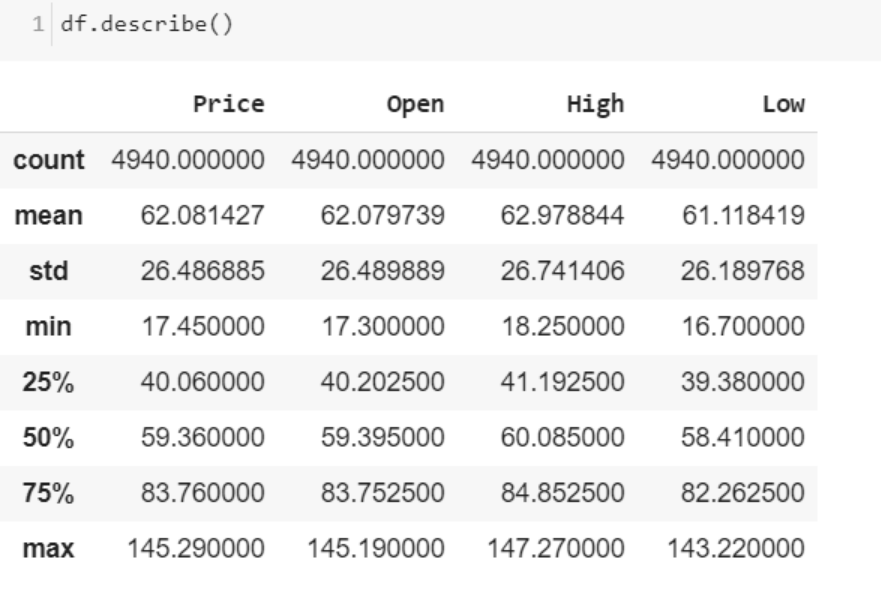# R语言ARMA-GARCH-COPULA模型和金融时间序列案例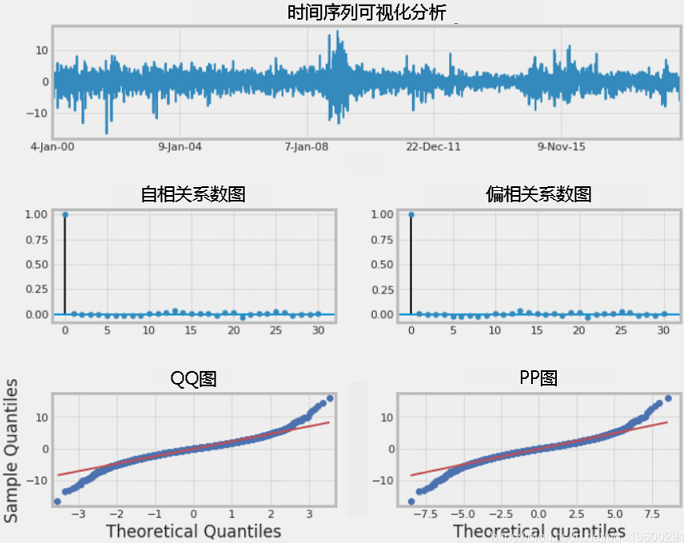### 随时关注您喜欢的主题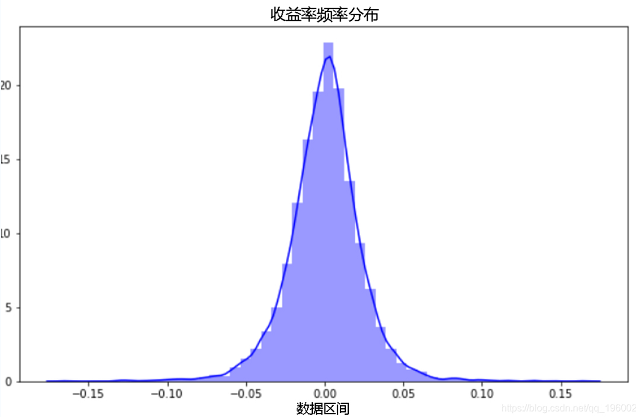`````` sns.distplot(df.returns, color=’blue’) #密度图

# 汇总统计数据
print(df.returns.describe()) ``````

Jarque-Bera检验的值表明，传统的正态分布假设不适用于原油收益的真实分布。

``````ADF = ADF(df.returns)

kpss = KPSS(df.returns)
print(kpss.summary().as_text()) ``````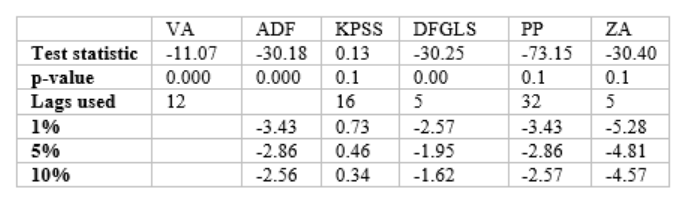# 非线性动力学

`````` # 计算最近价格的Hurst系数

tau = [sqrt(std(subtract(closes_recent[lag:], closes_recent[:-lag]))) for lag in lags]
m = polyfit(log(lags), log(tau), 1)
hurst = m*2 ``````

# H_（0.531）表示具有长期依赖性的随机运动时间序列。证明了 本研究中GARCH模型的合理性 。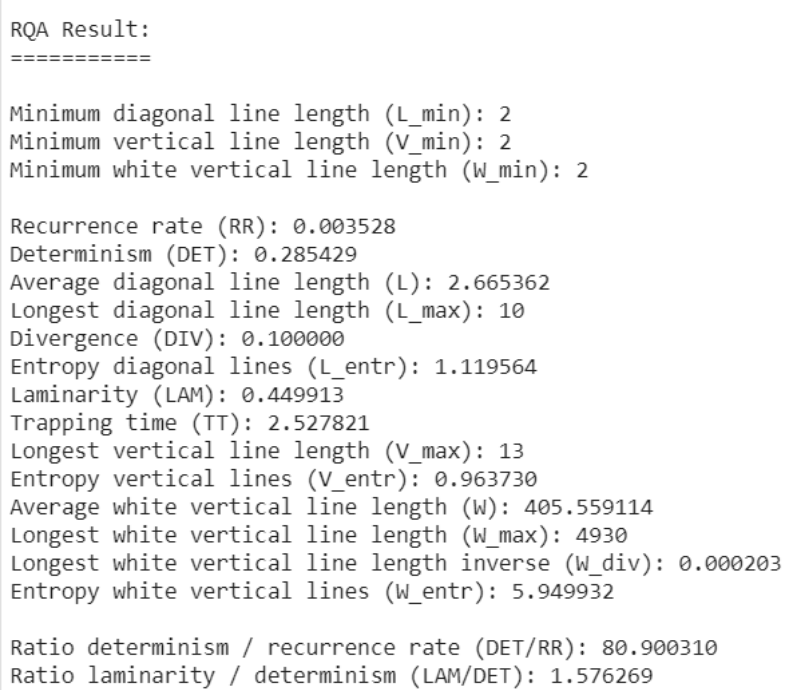`````` computation = RQAComputation.create(settings,
verbose=True)
result = computation.run()
result.min_diagonal_line_length = 2 ``````

# GARCH模型

`` X = 100* df.returns ``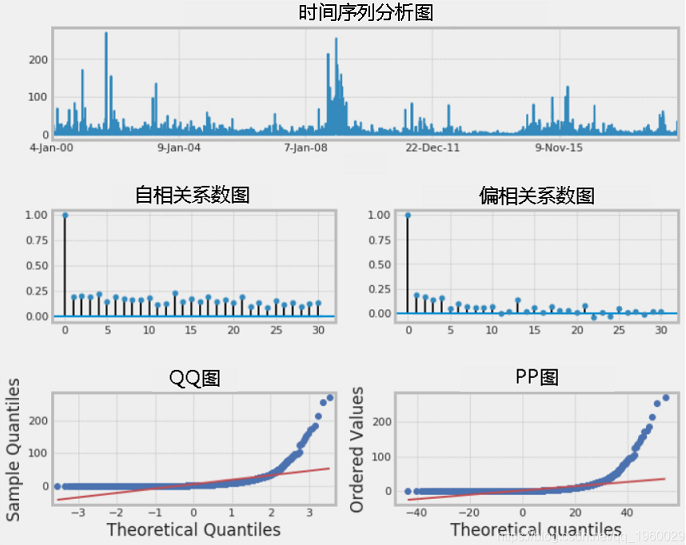`````` def getbest(TS):
best_aic = np.inf

for i in pq_rng:
for d in d_rng:
for j in pq_rng:
try:
tmp_mdl = smt.ARIMA(TS, order=(i,d,j)).fit(

#aic: 22462.01 | order: (2, 0, 2)``````
``````gam = arch_model(Model.resid, p=2, o=0, q=2, dist=’StudentsT’)
gres = gam.fit(update_freq=5, disp=’off’)
print(gres.summary())

tsplot(gres.resid**2, lags=30)``````

# 我们可以看到平方残差具有自相关的依据。让我们拟合一个GARCH模型并查看其性能。我将按照以下步骤进行操作：

• 通过ARIMA（p，d，q） 模型的组合进行迭代 以拟合最优时间序列。
• 根据 具有最低AIC的ARIMA模型选择 GARCH模型 。
• 将 GARCH（p，q） 模型拟合到时间序列。
• 检查模型残差和平方残差进行自相关

`` arch_model(X, p=2, q=2, o=1,power=2.0, vol=’Garch’, dist=’StudentsT’) ``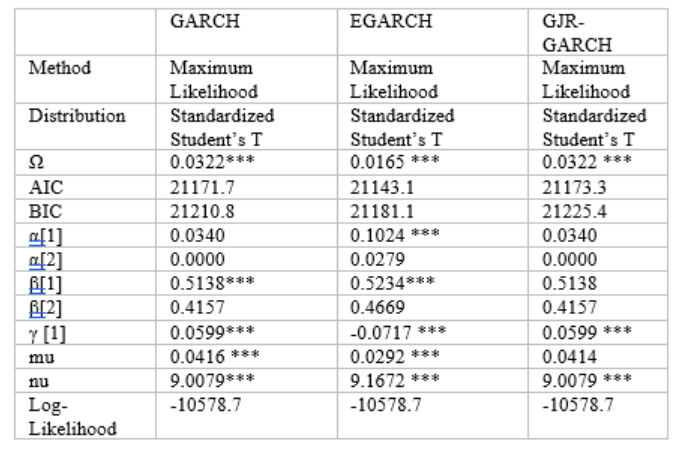`` am = arch_model(X, p=2, q=2, o=1,power=2.0, vol=’Garch’, dist=’StudentsT’) ``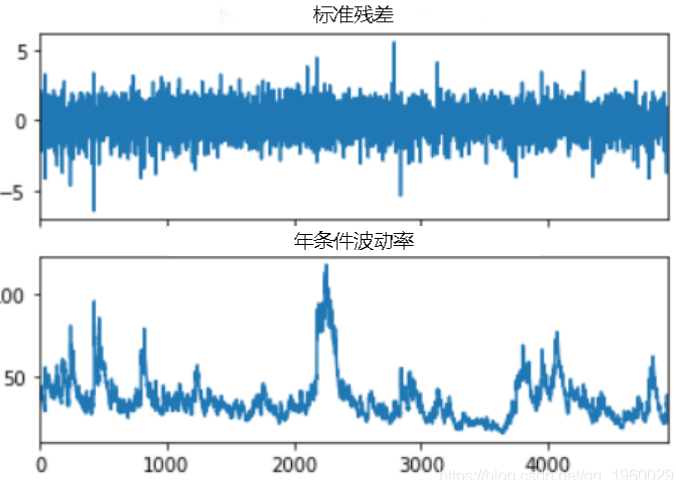``````std_resid =  resid /  conditional_volatility
unit_var_resid =  resid /  resid.std()``````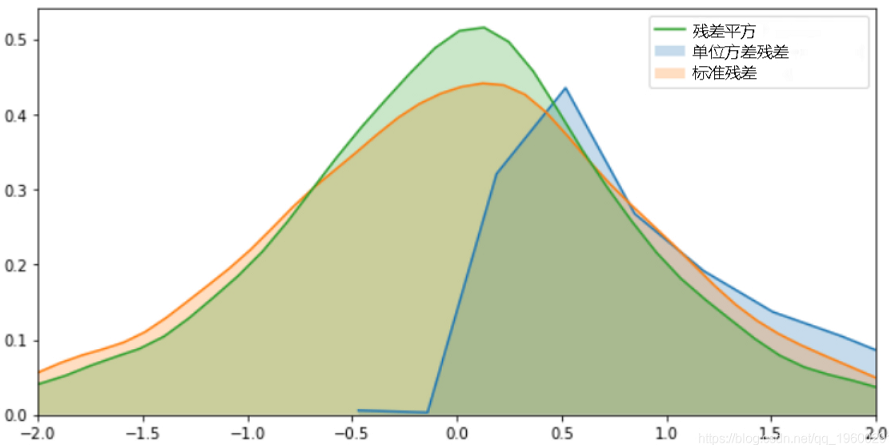`````` sns.kdeplot(squared_resid, shade=True)

# 标准化残差和条件波动图显示了一些误差，但幅度不大。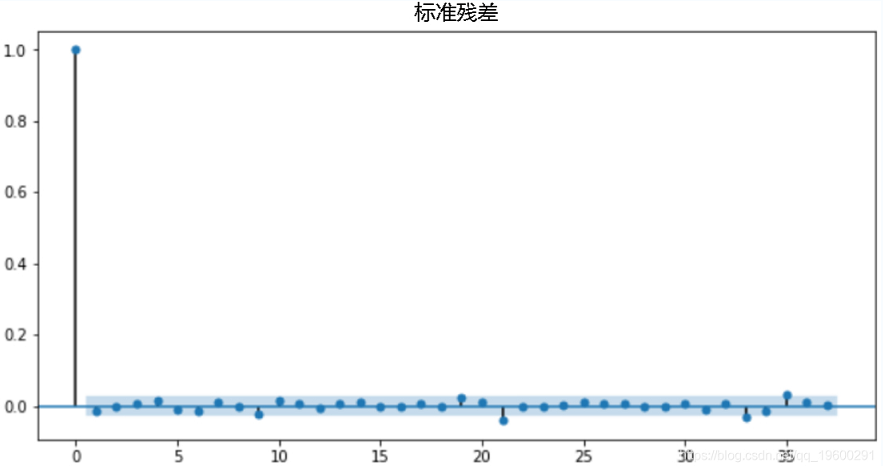``plot_acf(std_resid) ``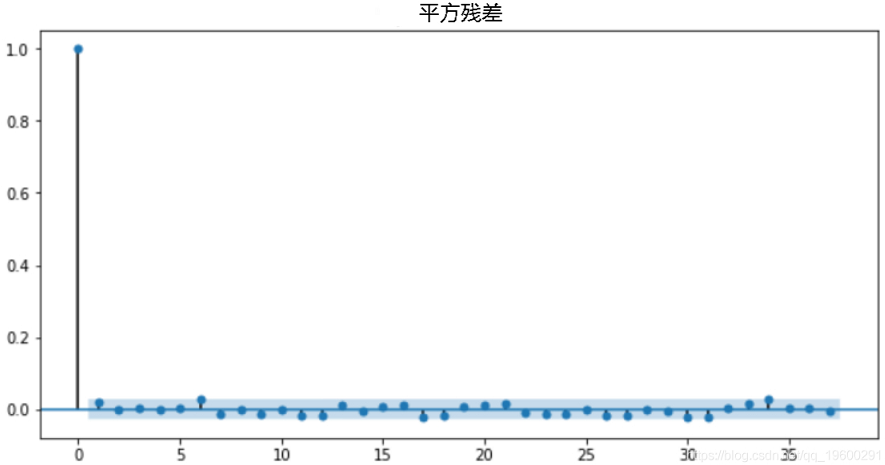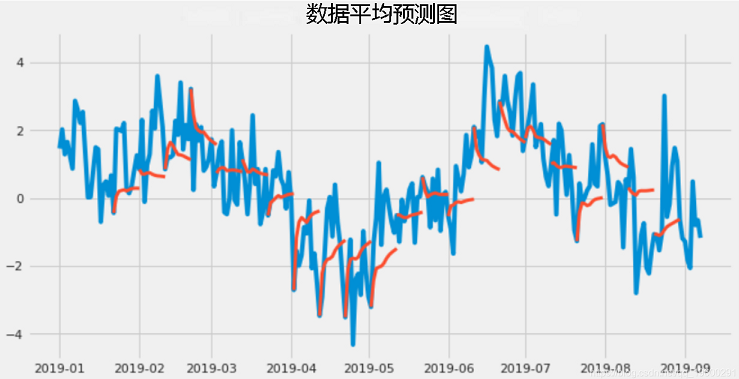`````` res = am.fit()
fig = res.hedgehog_plot(type=’mean’)``````

# 基于模拟的预测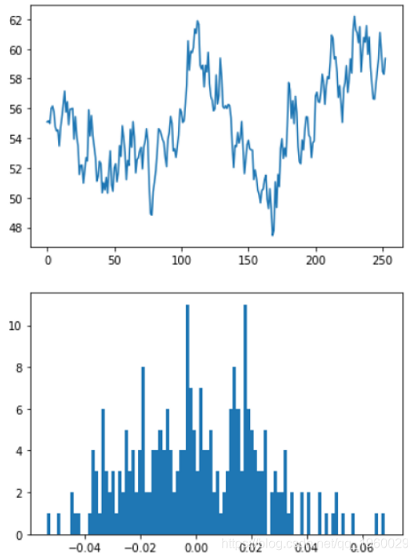`````` #定义变量
T = 252 #交易天数
mu = 0.0622 #收益
vol = 0.3737 #波动率

daily_returns=np.random.normal((1+mu)**(1/T),vol/sqrt(T),T)

#生成图-价格序列和每日收益的直方图
plt.plot(price_list)
plt.hist(daily_returns-1, 100) ``````

`````` #设置一个空列表来保存我们每个模拟价格序列的最终值
result = []

S = df.Price[-1] #起始股票价格（即最​​后可用的实际股票价格）
T = 252 #交易天数
mu = 0.0622 #收益率
vol = 0.3737 #波动率

#选择要模拟的运行次数-我选择了10,000
for i in range(10000):
#使用随机正态分布创建每日收益表
daily_returns= np.random.normal((1+mu)**(1/T),vol/sqrt(T),T)

#设定起始价格，并创建由上述随机每日收益生成的价格序列

#将每次模拟运行的结束值添加到我们在开始时创建的空列表中
result.append(price_list[-1]) ``````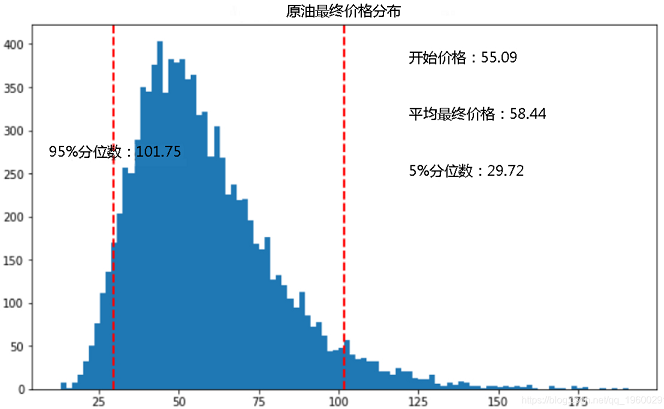# 概括

Kaizong Ye拓端研究室（TRL）的研究员。

​非常感谢您阅读本文，如需帮助请联系我们！QQ在线咨询

15121130882

0571-63341498

## 关注有关新文章的微信公众号

This will close in 0 seconds